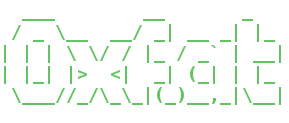Level 34

by Joel I

The numbers below are determined by the following function:

```function Y(int x, int k1, int k2):
return pow(x + 2, k1) mod (k1-2)*(k2-2)```

Sequences A, B, C, and D each use different values for K1 and K2, all random integers between 1000 and 1200. The first three values are given. (Y(1), Y(2), Y(3))

The solution to this level is the sum of the next numbers in the seqeunce for A, B, C, and D.

 842809,325416,215065 871425,106720,184705 802161,1069696,1120195 819327,997768,520985

You have 60 seconds to solve this level